# 【C++初阶】一、C++入门（万字总结）|关于C++ |命名空间 |C++ 输入&输出 |缺省参数 |函数重载 |引用 |内联函数 |auto关键字 |基于范围的for循环 |指针空值nullptr

1.1  什么是C++ ?

1.2  C++ 发展史

二、C++关键字(C++98)

三、命名空间

3.1  命名空间出现的原因

3.2  命名空间的定义

3.2.1  正常的命名空间定义

3.3.2  命名空间的使用

3.3.3  使用命名空间的一些建议

5.1缺省参数的概念

5.2  缺省参数分类

5.2.1  全缺省参数

​5.2.2  半缺省参数

6.1  函数重载概念

6.2  C++支持函数重载的原理--名字修饰(name Mangling)

6.3  extern “C”

7.1  引用概念

7.2  引用特性

7.2.1  引用在定义时必须初始化

7.2.2   一个变量可以有多个引用

7.2.3  引用一旦引用一个实体，再不能引用其他实体

7.3  常引用

7.4  引用使用场景

7.4.1  引用做参数

7.4.2  引用做返回值

7.5  引用和指针的区别

8.1  内联函数概念

8.2  内联函数的特性

九、auto关键字(C++11)

9.1 auto简介

9.2  auto的使用细则

9.2.1  auto与指针和引用结合起来使用

9.2.2  在同一行定义多个变量

9.3  auto不能推导的场景

9.3.1  auto不能作为函数的参数

9.3.2  auto不能直接用来声明数组

9.3.3

9.3.4

10.1  范围for的语法

10.2  范围for的使用条件

10.2.1  for循环迭代的范围必须是确定的

10.2.2  迭代的对象要实现 ++ 和 == 的操作

11.1  C++98中的指针空值

11.2  C++11中的指针空值nullptr

# 一、关于C++

## 1.1  什么是C++ ?

C 语言是结构化和模块化的语言，适合处理较小规模的程序。对于复杂的问题，规模较大的程序，需要高度的抽象和建模时，C 语言则不合适。为了解决软件危机， 20世纪80年代， 计算机界提出了 OOP(objectoriented programming：面向对象)思想，支持面向对象的程序设计语言应运而生。
1982年，Bjarne Stroustrup 博士在 C 语言的基础上引入并扩充了面向对象的概念，发明了一种新的程序语言。为了表达该语言与C语言的渊源关系，命名为 C++ 。因此：C++ 是基于C语言而产生的，它既可以进行 C 语言的过程化程序设计，又可以进行以抽象数据类型为特点的基于对象的程序设计，还可以进行面向对象的程序设计。

C语言与 C++ 的区别和联系：C语言是面向过程的语言，而 C++ 是面向对象的语言。从语法上讲，C语言是 C++ 的一部分，C语言代码几乎不用修改就能够用 C++ 的方式编译，简单说就是 C++ 兼容 C语言。

## 1.2  C++ 发展史

1979年，贝尔实验室的本贾尼等人试图分析unix内核的时候，试图将内核模块化，于是在 C 语言的基础上进行扩展，增加了类的机制，完成了一个可以运行的预处理程序，称之为 C with classes

语言的发展就像是练功打怪升级一样，也是逐步递进，由浅入深的过程。我们先来看下 C++ 的历史版本。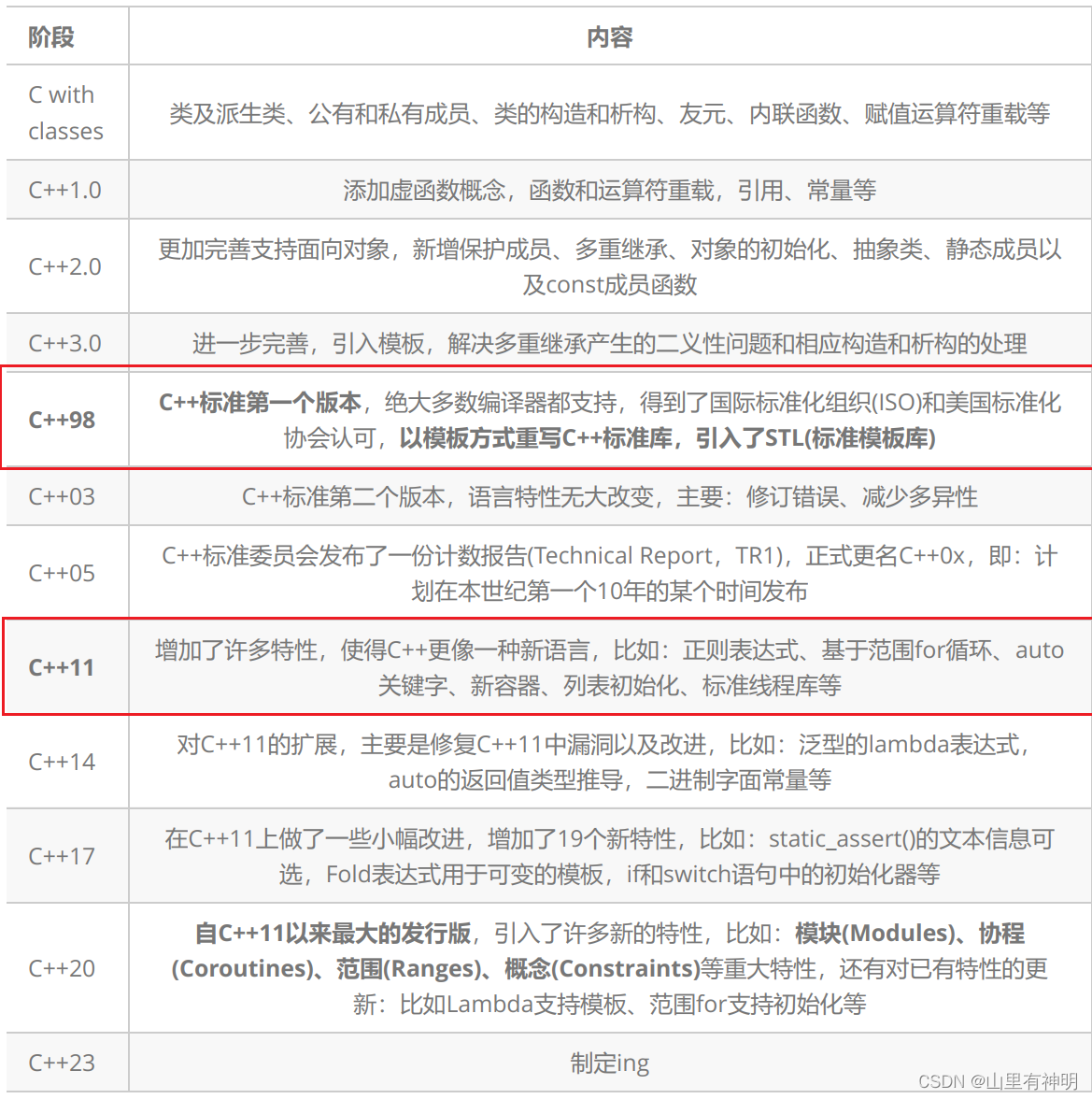C++ 还在不断的向后发展。但是：现在公司主流使用还是 C++98 和 C++11

# 二、C++关键字(C++98)

C++ 总计 63 个关键字，C语言 32 个关键字。如图：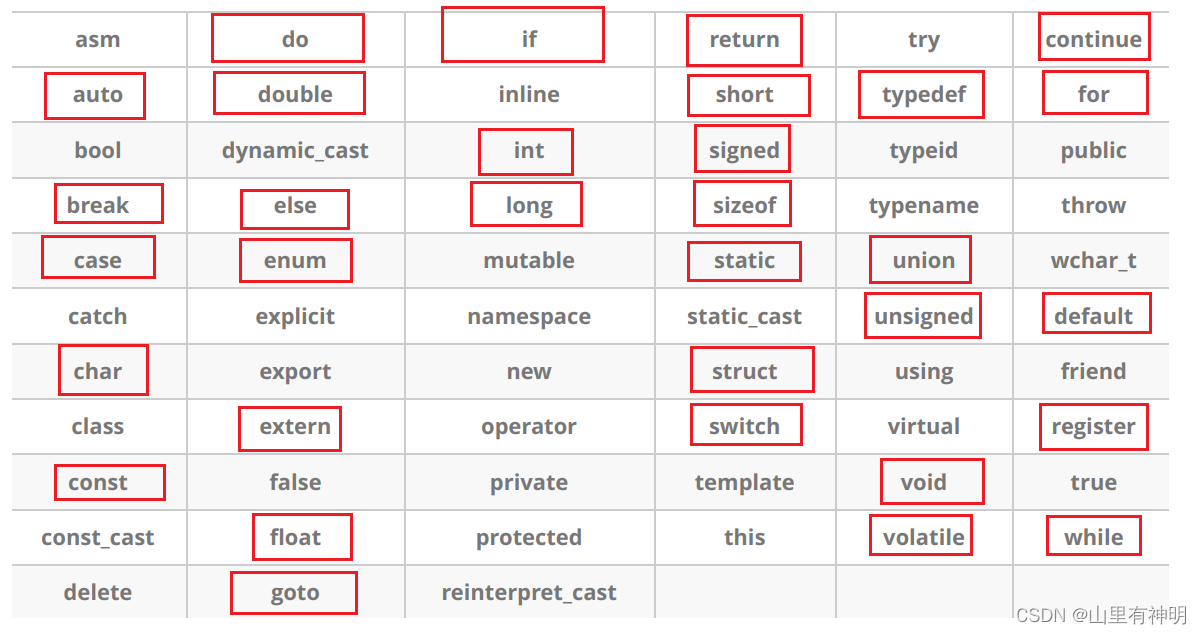打红色方框的是 C语言的关键字，剩下的是  C++ 对 C语言关键字的补充。

# 三、命名空间

## 3.1  命名空间出现的原因

在 C/C++ 中，变量、函数和后面要学到的类都是大量存在的，这些变量、函数和类的名称将都存在于全局作用域中，可能会导致很多冲突。使用命名空间的目的是对标识符的名称进行本地化，以避免命名冲突或名字污染，namespace关键字的出现就是针对这种问题的。

``````#include <stdio.h>
#include <stdlib.h>
int rand = 10;
int main()
{
printf("%d\n", rand);
return 0;
}``````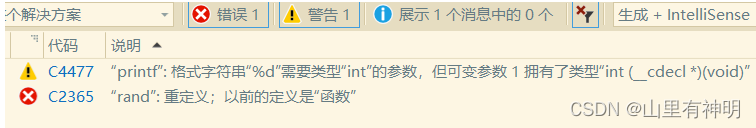C语言没办法解决类似这样的命名冲突问题，所以 C++ 提出了 namespace 来解决。

## 3.2  命名空间的定义

定义命名空间，需要使用到 namespace 关键字，后面跟命名空间的名字，然后接一对 {} 即可，{} 中的内容即为命名

### 3.2.1  正常的命名空间定义

（1）定义

``````//namespace 后加命名空间的名字，例如我将它命名为 fengye
namespace fengye
{
//注意：一个命名空间就定义了一个新的作用域，命名空间中的所有内容都局限于该命名空间中
//命名空间中可以定义变量/函数/类型
int rand = 10;
{
return a + b;
}
struct Node
{
struct Node* next;
int val;
};``````

（2）命名空间可以嵌套

``````namespace fengye1
{
int a;
int b;
{
return a + b;
}
namespace fengye2
{
int c;
int d;
int Sub(int c, int d)
{
return c - d;
}
}
}``````

（3）合并命名空间

同一个工程中允许存在多个相同名称的命名空间,编译器最后会合成同一个命名空间中。

ps：一个工程中的 Test.h 和 Test.cpp 中两个 命名空间fengye 会被合并成一个。

``````//Test.h
namespace fengye
{
int scanf = 1;
int fun(int a, int b)
{
return a * b;
}
}
//Test.cpp
namespace fengye
{
int rand = 10;
{
return a + b;
}
struct Node
{
struct Node* next;
int val;
};
}``````

### 3.3.2  命名空间的使用

（1）加命名空间名称及作用域限定

符号 “:: ”在 C++ 中叫做作用域限定符，通过“命名空间名称::命名空间成员”便可以访问到命名空间中相应的成员。

ps:

``````#include <stdio.h>
#include <stdlib.h>
namespace fengye
{
int rand = 10;
}
int main()
{
printf("%d\n", rand);//没有作用域限定符
printf("%p\n", rand);
printf("%d\n", fengye::rand);//添加作用域限定符
return 0;
}``````

（2）使用 using 将命名空间中某个成员引入

using 是 C++ 中的一个关键字，该关键字的作用是展开命名空间代码中的 using fengye::rand 指的是把 rand 单独展开在全局域中，展开之后的 rand 就相当于一个没有被封装的全局变量，可以任何调用。

ps:

``````#include <stdio.h>
#include <stdlib.h>
namespace fengye
{
int a = 10;
}

using fengye::rand;

int main()
{
printf("%d\n", a);
return 0;
}``````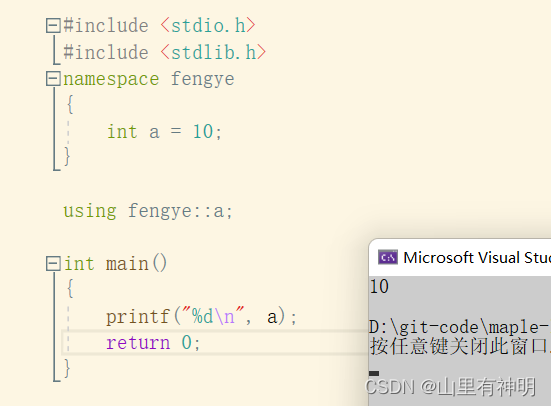但是如果展开的是一个已经定义有的函数，会造成重定义。因为展开之后的 rand 就相当于一个没有被封装的全局变量，相当于定义了 int rand = 10，就会造成与库里的函数名字相冲突，就会造成重定义。

ps：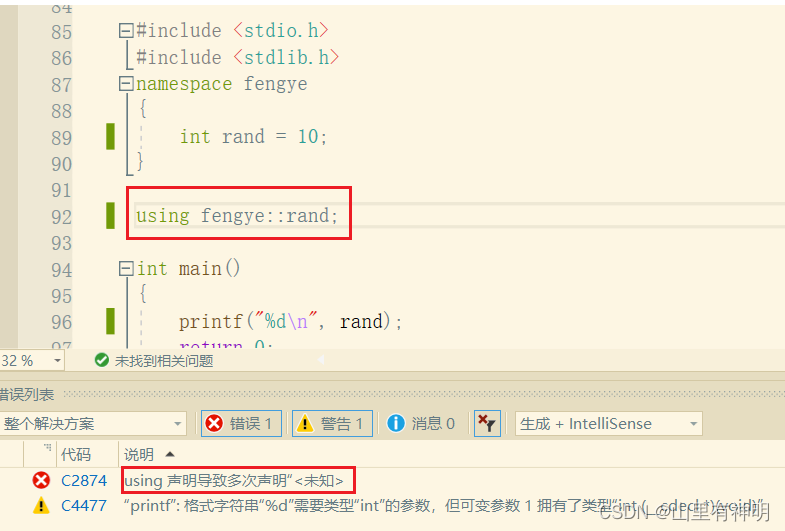（3）使用using namespace 命名空间名称引入

using namespace + 命名空间的名字 （ps：using namespace fengye），相当于将命名空间 fengye 利的变量全部展开到全局域中，这样域中的变量可以直接调用，变量前面不用加 fengye::

ps：

``````#include <stdio.h>
namespace fengye
{
int a = 10;
int b = 20;
int c = 30;
}

using namespace fengye;

int main()
{
printf("%d\n", a);
printf("%d\n", b);
printf("%d\n", c);
return 0;
}``````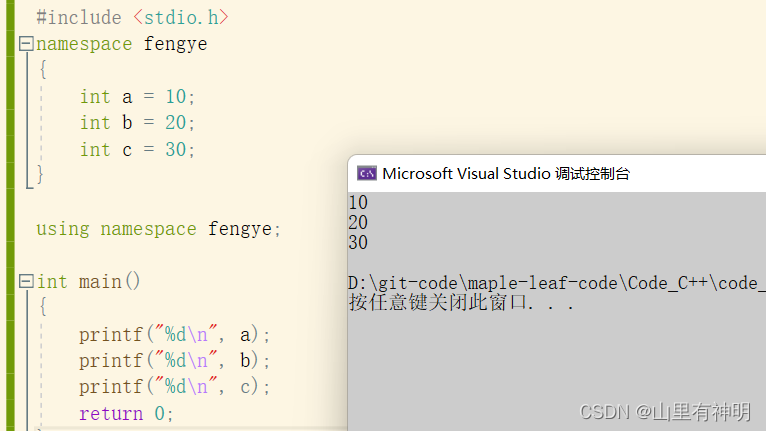• using namespace std

std 是C++标准库的命名空间名，C++ 将标准库的定义实现都放到这个命名空间中.
using namespace std 把库里的所有东西全部展开到全局域了， 这样自己定义的东西可能就会与库中的造成冲突，所以工程项目中是不推荐使用这种方式的，日常练习可以使用，比较方便。

### 3.3.3  使用命名空间的一些建议

1. 项目中，尽量不要 using namespace std;
2. 日常练习用 using namespace std；
3. using namespace std 展开，标准库就全部暴露出来了，如果我们定义跟库重名的类型/对象/函数，就存在冲突问题。该问题在日常练习中很少出现，但是项目开发中代码较多、规模大，就很容易出现。所以建议在项目开发中使用，像std::cout这样使用时指定命名空间 + using std::cout展开常用的库对象/类型等方式。

# 四、C++ 输入&输出

ps：

``````#include<iostream>
using namespace std;
int main()
{
cout << "Hello world!!!" << endl;
return 0;
}``````

运行结果：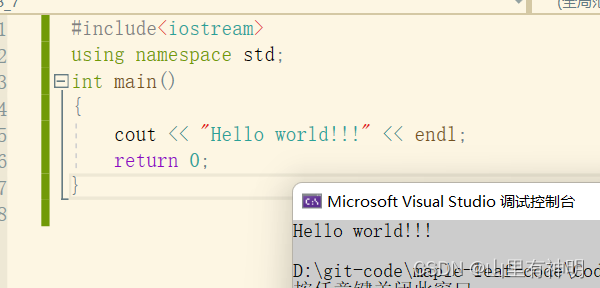1. 使用 cout 标准输出对象(控制台)和 cin 标准输入对象(键盘)时，必须包含 < iostream > 头文件以及按命名空间使用方法使用std。
2.  cout 和 cin 是全局的流对象，endl 是特殊的C++符号，表示换行输出，他们都包含在包含 < iostream > 头文件中。
3.  << 是流插入运算符，>> 是流提取运算符。
4. 使用C++输入输出更方便，不需要像 printf/scanf 输入输出时那样，需要手动控制输入输出格式。C++ 的输入输出可以自动识别变量类型。
5. 关于cout和cin还有很多更复杂的用法，比如控制浮点数输出精度，控制整形输出进制格式等等。因为C++兼容C语言的用法，为了方便一些可以直接使用 C语言的格式控制符。

# 五、缺省参数

## 5.1缺省参数的概念

缺省参数是声明或定义函数时为函数的参数指定一个缺省值。在调用该函数时，如果没有指定实参则采用该形参的缺省值，否则使用指定的实参。

ps：

``````#include<iostream>
using namespace std;
void Func(int a = 0)
{
cout << a << endl;
}

int main()
{
Func(); // 没有传参时，使用参数的默认值，C++有了缺省参数可以不传参
Func(10); // 传参时，使用指定的实参
return 0;
}``````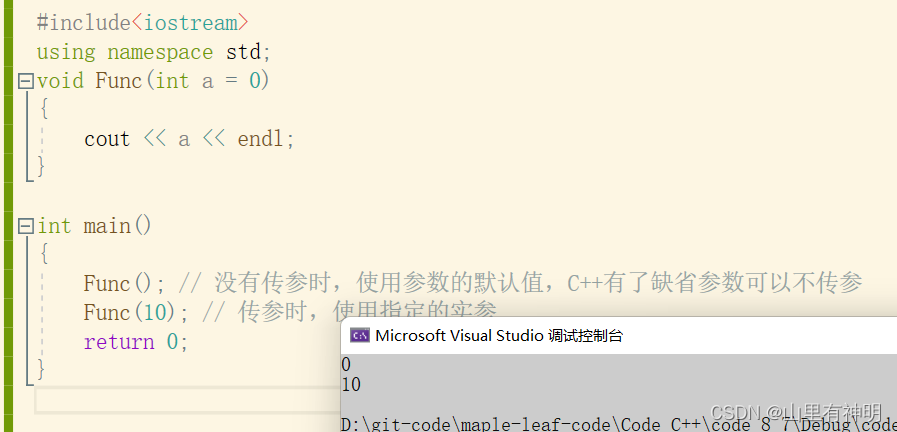## 5.2  缺省参数分类

### 5.2.1  全缺省参数

ps：

``````#include<iostream>
using namespace std;
void Func(int a = 10, int b = 20, int c = 30)
{
cout << "a = " << a << ' ';
cout << "b = " << b << ' ';
cout << "c = " << c << endl;
}

int main()
{
Func(); // 没有传参时，使用参数的默认值
// 传参时，使用指定的实参
Func(1); //支持
Func(1, 2);//支持
Func(1, 2, 3);
return 0;
}``````

###5.2.2  半缺省参数

ps：

``````#include<iostream>
using namespace std;
void Func(int a, int b = 10, int c = 20)
{
cout << "a = " << a << ' ';
cout << "b = " << b << ' ';
cout << "c = " << c << endl;
}

int main()
{
Func(1); //支持，从左往右给
Func(1, 2);//支持
Func(1, 2, 3);//支持
//Func(, 2, );//不支持
//Func(, , 3);//不支持
return 0;
}``````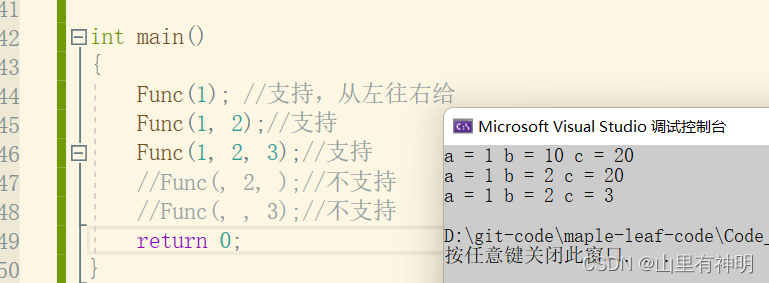注意

1. 半缺省参数必须从右往左依次来给出，不能间隔着给
2. 缺省参数不能在函数声明和定义中同时出现
3. 缺省值必须是常量或者全局变量
4.  C语言不支持（编译器不支持）

ps：

``````//2.缺省参数不能在函数声明和定义中同时出现
//Test.h
void Func(int a = 10);
//Test.cpp
void Func(int a = 20)
{}
// 注意：如果生命与定义位置同时出现，恰巧两个位置提供的值不同，那编译器就无法确定到底该用那个缺省值。``````

# 六、函数重载

## 6.1  函数重载概念

函数重载是函数的一种特殊情况，C++允许在同一作用域中声明几个功能类似的同名函数这些同名函数

，常用来处理实现功能类似数据类型不同的问题。

ps：

``````#include<iostream>
using namespace std;
// 1、参数类型不同
{
cout << "int Add(int left, int right)" << endl;
return left + right;
}
{
cout << "double Add(double left, double right)" << endl;
return left + right;
}
// 2、参数个数不同
void f()
{
cout << "f()" << endl;
}
void f(int a)
{
cout << "f(int a)" << endl;
}
// 3、参数类型顺序不同
void f(int a, char b)
{
cout << "f(int a,char b)" << endl;
}
void f(char b, int a)
{
cout << "f(char b, int a)" << endl;
}
int main()
{
//1、参数类型不同
// 2、参数个数不同
f();
f(10);
//3、参数类型顺序不同
f(10, 'a');
f('a', 10);

return 0;
}``````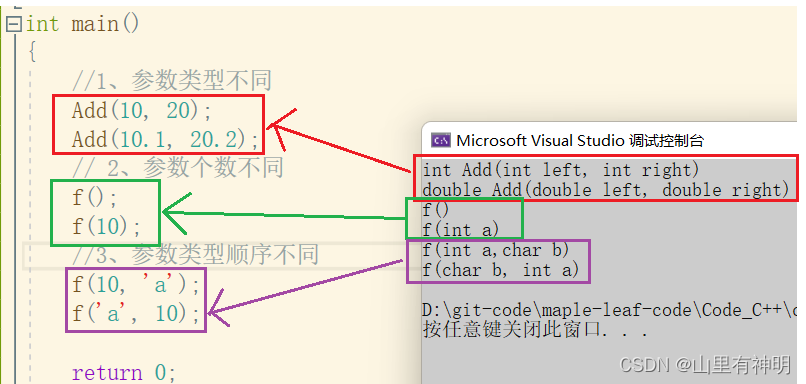• 若函数只有返回值不懂，其他相同，不构成函数重载

## 6.2  C++支持函数重载的原理--名字修饰(name Mangling)

1.  采用C语言编译器编译后结果：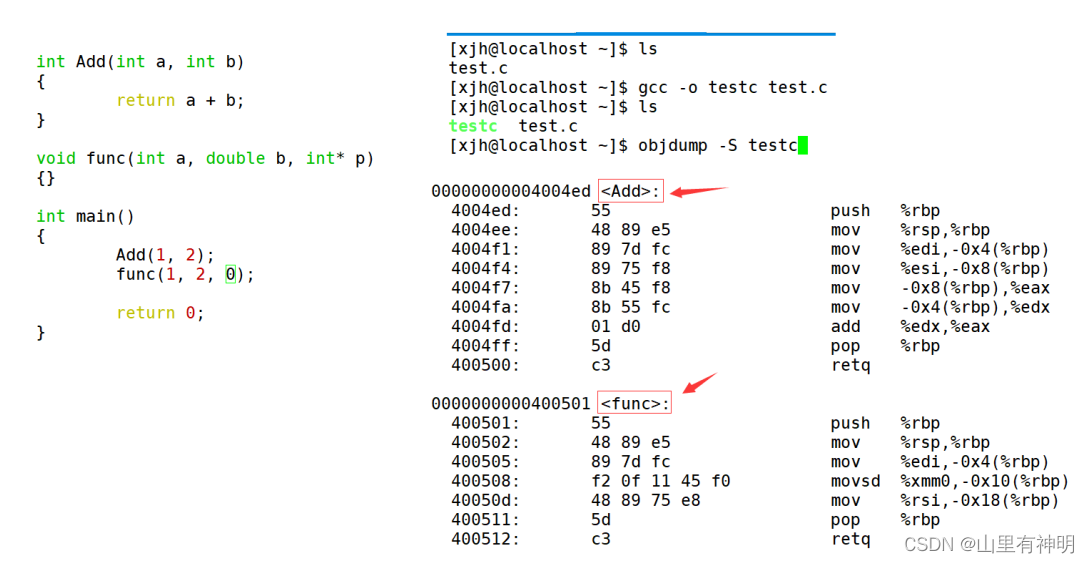结论：在linux下，采用gcc编译完成后，函数名字的修饰没有发生改变。

2.  采用C++编译器编译后结果结论：在linux下，采用g++编译完成后，函数名字的修饰发生改变，编译器将函数参数类型信息添加到修改后的名字中。

通过这里就理解了C语言没办法支持重载，因为同名函数没办法区分。而C++是通过函数修饰规则来区分，只要参数不同，修饰出来的名字就不一样，就支持了重载。

如果两个函数函数名和参数是一样的，返回值不同是不构成重载的，因为调用时编译器没办法区分。

## 6.3  extern “C”

1. C++中调用C语言实现的静态库或者动态库，反之亦然
2. 多人协同开发时，有些人擅长用C语言，有些人擅长用C++

# 七、引用

## 7.1  引用概念

引用不是新定义一个变量，而是给已存在变量取了一个别名，编译器不会为引用变量开辟内存空间，它和它引用的变量共用同一块内存空间

ps：

``````#include<iostream>
int main()
{
int a = 10;
int& ra = a;//<====定义引用类型,类型（int）& 引用变量名（ra） = 引用实体（a）
printf("%p\n", &a);
printf("%p\n", &ra);

return 0;
}``````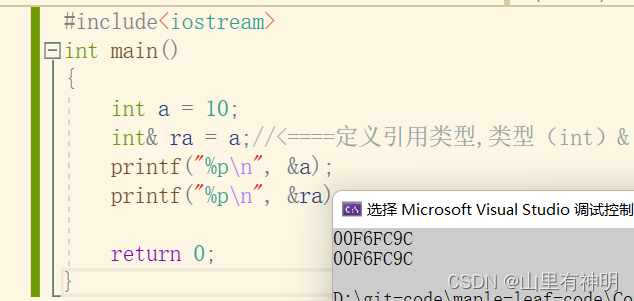``````//ps:
double d = 12.34;
//int& rd = d; // 该语句编译时会出错，类型不同``````

## 7.2  引用特性

### 7.2.1  引用在定义时必须初始化

ps：

``````#include<iostream>
int main()
{
int a = 10;
int& ra;//错误

return 0;
}``````### 7.2.2   一个变量可以有多个引用

ps：

``````#include<iostream>
using namespace std;
int main()
{
int a = 10;
int& ra = a;
int& rb = a;

cout << &a << endl;//取地址
cout << &ra << endl;
cout << &rb << endl;
return 0;
}``````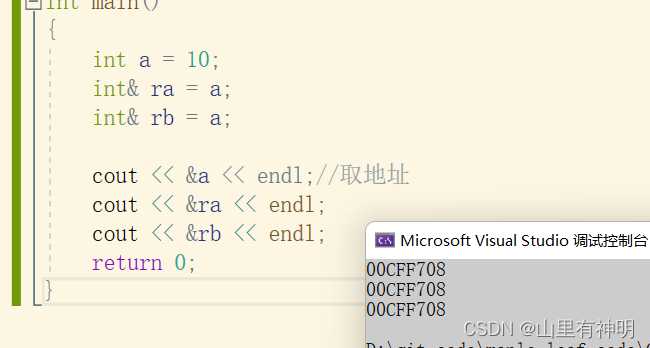### 7.2.3  引用一旦引用一个实体，再不能引用其他实体

ps：

``````#include<iostream>
using namespace std;
int main()
{
int a = 10;
int& ra = a;
int b = 20;
ra = b;// ra 是 b 的别名呢？还是 b 赋值给 ra 呢？答案是 b 赋值给 ra

cout << a << endl;
cout << ra << endl;
return 0;
}``````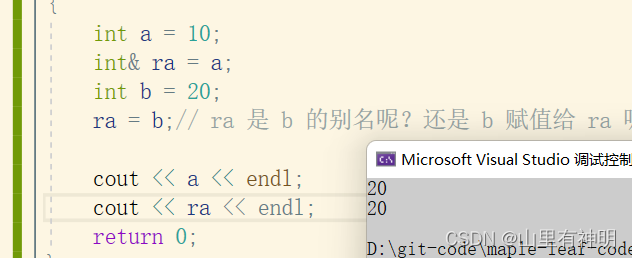结果说明 b 赋值给了 ra ，又因为 ra 是 a 的引用，所以 a 的值被改成了 20

## 7.3  常引用

定义：被 const 修饰的引用是常引用。常引用会涉及到权限的问题。

ps：

``````//权限不能放大
const int a = 10;
//int& ra = a;//error
const int& ra = a;//true

//权限可以缩小
int c = 10;
const int& rc = c;//true

//权限相同
const int d = 10;
const int& rd = d;
``````

ps：

``````double e = 12.34;
int f = e;//发生整型提升

//int& re = e; // 该语句编译时会出错，类型不同，本质是权限放大了
const int& re = e;//true``````

将浮点型变量 e 赋值给整型变量 f 时会发生隐式类型转换，中间会产生一个临时变量，这个临时变量具有常性，跟常变量的属性一致。ps：int& re = e，e 产生的临时变量具有常性，只有读权限，权限缩小了；而 re 具有读写权限，这样一来临时变量转换成int& re 后，临时变量的权限被放大了，所以 error。而 const 修饰的引用，权限变小了，与临时变量的权限一致，可以转换。

## 7.4  引用使用场景

### 7.4.1  引用做参数

ps：

``````#include<iostream>
using namespace std;
void Swap(int& a1, int& a2)
{
int temp = a1;
a1 = a2;
a2 = temp;
}

int main()
{
int a = 1, b = 2;
Swap(a, b);
cout << "a = " << a << endl;
cout << "b = " << b << endl;
return 0;
}``````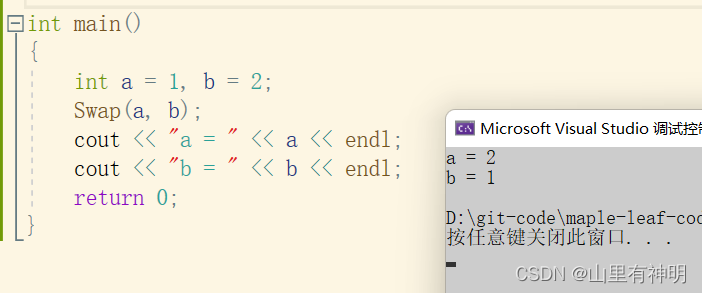1. 做输出型参数
2. 大对象传参提高效率

### 7.4.2  引用做返回值

引用也能做返回值，但是要特别注意，返回的数据不能是函数内部创建的局部变量，因为在函数内部定义的局部变量会随着函数调用的结束而被销毁。返回的数据必须是被 static 修饰或者是动态开辟的或者是全局变量等不会随着函数调用的结束而被销毁的数据。

ps：

``````#include<iostream>
using namespace std;
int& Count()
{
int n = 0;
n++;
return n;//n 随着函数调用结束就被销毁了
}

int main()
{
int& ret = Count();
cout << ret << endl;
cout << ret << endl;

return 0;
}``````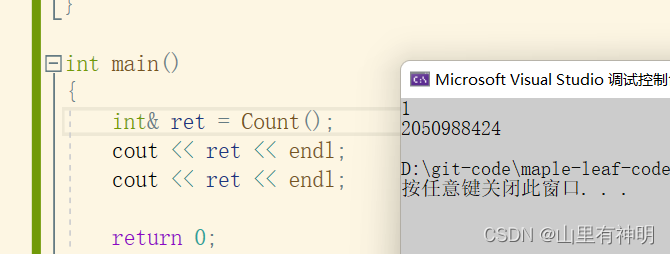1. 函数运行时，系统需要给该函数开辟独立的栈空间，用来保存该函数的形参、局部变量以及一些寄存器信息等
2. 函数运行结束后，该函数对应的栈空间就被系统回收了
3. 空间被回收指该块栈空间暂时不能使用，但是内存还在;比上课要申请教室，上完课之后教室归还给学校，但是教室本身还在，不能说归还了之后，教室就没有了

``static int n = 0;``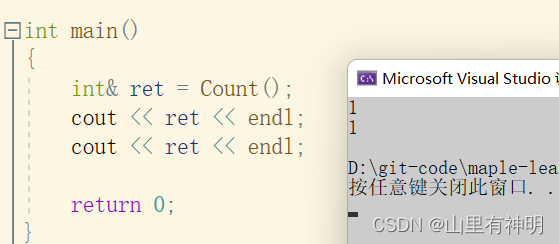注意：如果函数返回时，出了函数作用域，如果返回对象还在(还没还给系统)，则可以使用引用返回，如果已经还给系统了，则必须使用传值返回。

1. 输出型返回对象，调用者可以修改返回对象
2. 减少拷贝，提高效率

## 7.5  引用和指针的区别

ps：

``````#include<iostream>
using namespace std;
int main()
{
int a = 10;
int& ra = a;
cout << "&a = " << &a << endl;
cout << "&ra = " << &ra << endl;
return 0;
}``````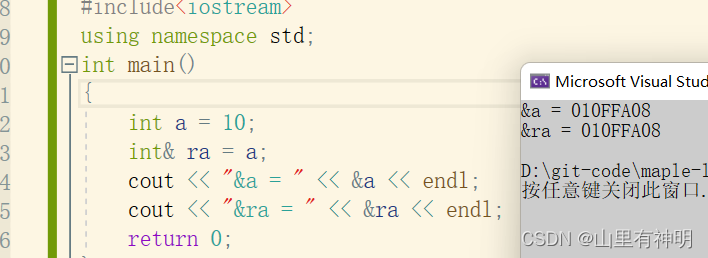在底层实现上，引用是按照指针方式来实现的。

ps：

``````#include<iostream>
using namespace std;
int main()
{
//从语法角度，ra没有开辟空间
int a = 10;
int& ra = a;
ra = 20;
//从语法角度，pa 开了4 or 8 byte
int* pa = &a;
*pa = 20;
return 0;
}``````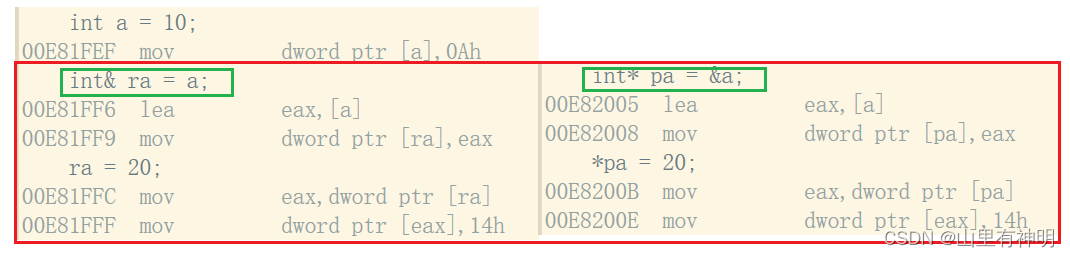左边引用和右边指针的反汇编代码进行对比，明显发现引用的底层就是用指针实现的。

1. 引用概念上定义一个变量的别名，指针存储一个变量地址。
2. 引用在定义时必须初始化，指针没有要求
3. 引用在初始化时引用一个实体后，就不能再引用其他实体，而指针可以在任何时候指向任何一个同类型实体
4. 没有NULL引用，但有NULL指针
5. 在sizeof中含义不同：引用结果为引用类型的大小，但指针始终是地址空间所占字节个数(32位平台下占4个字节)
6. 引用自加即引用的实体增加1，指针自加即指针向后偏移一个类型的大小
7. 有多级指针，但是没有多级引用
8. 访问实体方式不同，指针需要显式解引用，引用编译器自己处理
9. 引用比指针使用起来相对更安全

# 八、内联函数

## 8.1  内联函数概念

以 inline 修饰的函数叫做内联函数，编译时C++编译器会在调用内联函数的地方展开没有函数调用建立栈帧的开销，内联函数提升程序运行的效率。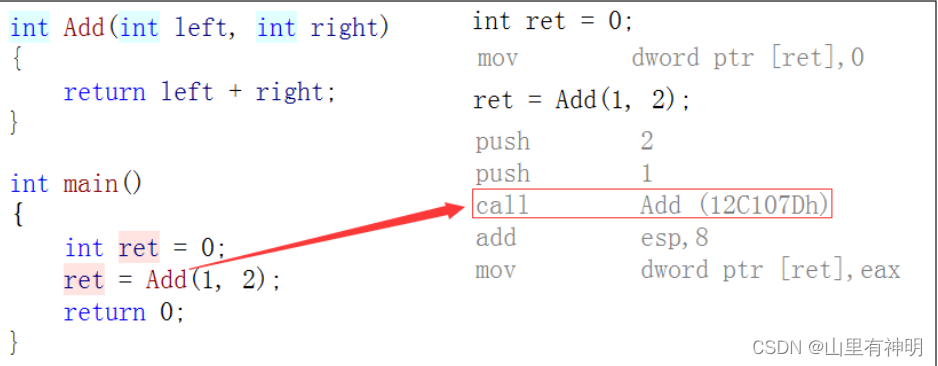如果在上述函数前增加i nline 关键字将其改成内联函数，在编译期间编译器会用函数体替换函数的调用，ps：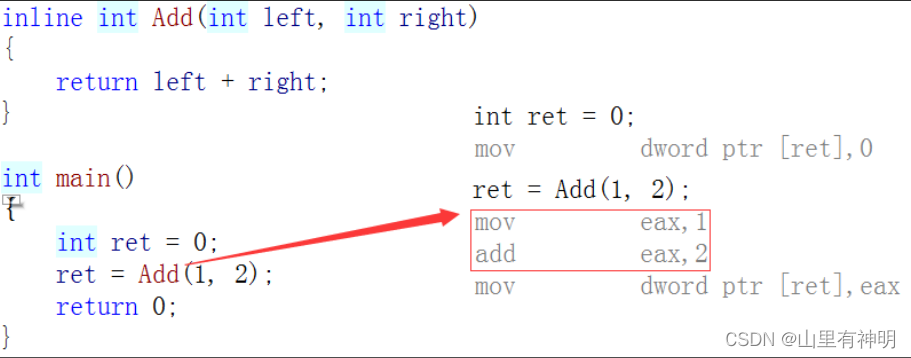查看方式：
2. 在debug模式下，需要对编译器进行设置，否则不会展开(因为 debug 模式下，编译器默认不会对代码进行优化)

vs2019 设置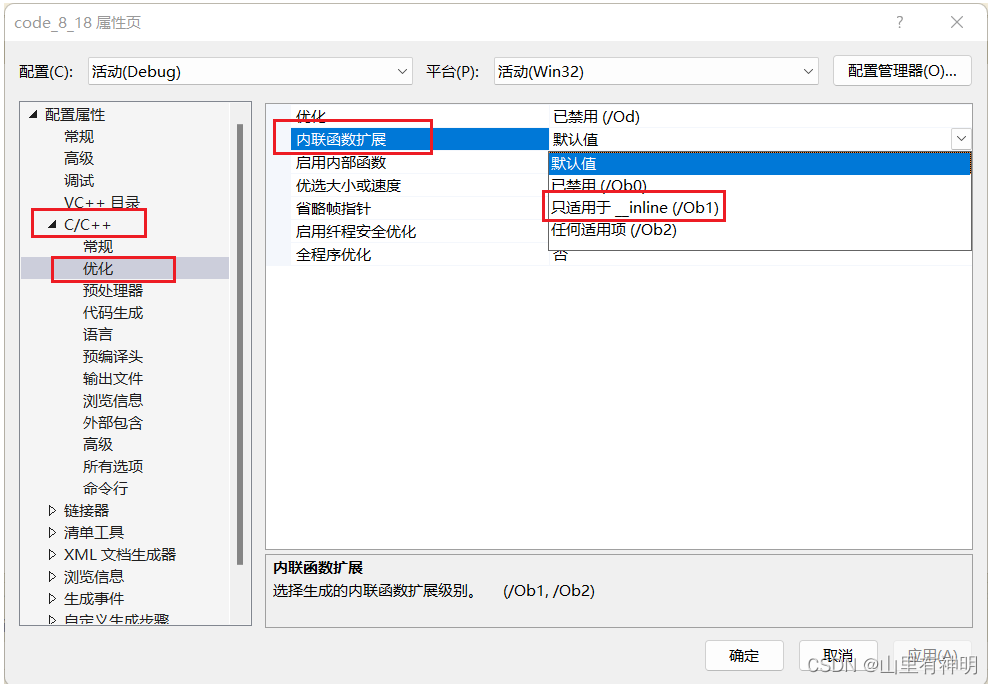## 8.2  内联函数的特性

1. inline是一种以空间换时间的做法，如果编译器将函数当成内联函数处理，在编译阶段，会用函数体替换函数调用，就是在函数调用的地方直接把内联函数展开。缺陷：可能会使目标文件变大，优势：少了调用开销，提高程序运行效率。

2. inline对于编译器而言只是一个建议，不同编译器关于inline实现机制可能不同，一般建议：将函数规模较小(即函数不是很长，具体没有准确的说法，取决于编译器内部实现)、不是递归、频繁调用的函数采用 inline 修饰，否则编译器会忽略 inline 特性。

3. inline不建议声明和定义分离，分离会导致链接错误。因为inline被展开，就没有函数地址了，链接就会找不到。
4. 宏定义是没有类型检查的，无论对还是错都是直接替换，而内联函数在编译时进行安全检查

ps：

``````3. inline不建议声明和定义分离，分离会导致链接错误。因为inline被展开，就没有函数地址了，链接就会找不到。
// Test.h
#include <iostream>
using namespace std;
inline void func(int i);

// Test.cpp
#include "Test.h"
void func(int i)
{
cout << i << endl;
}

// main.cpp
#include "Test.h"
int main()
{
func(10);
return 0;
}
// 链接错误：main.obj : error LNK2019: 无法解析的外部符号 "void __cdecl f(int)" (?
f@@YAXH@Z)，该符号在函数 _main 中被引用``````

# 九、auto关键字(C++11)

## 9.1 auto简介

在早期C/C++中auto的含义是：使用auto修饰的变量，是具有自动存储器的局部变量，但遗憾的是一直没有人去使用它。
C++11中，标准委员会赋予了auto全新的含义即：auto不再是一个存储类型指示符，而是作为一个新的类型指示符来指示编译器，auto声明的变量必须由编译器在编译时期推导而得。

ps：

``````#include<iostream>
using namespace std;
int TestAuto()
{
return 10;
}
int main()
{
int a = 10;
auto b = a;//auto 自动推导类型
auto c = 'a';
auto d = TestAuto();
//typeid().name 的作用是输出变量的类型
cout << typeid(b).name() << endl;
cout << typeid(c).name() << endl;
cout << typeid(d).name() << endl;
//auto e; 无法通过编译，使用auto定义变量时必须对其进行初始化
return 0;
}``````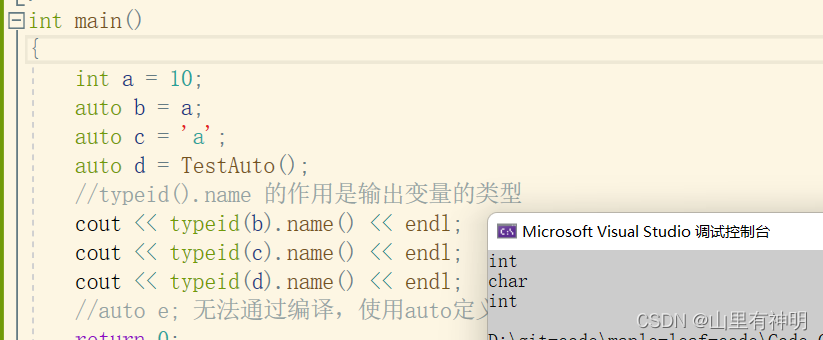使用auto定义变量时必须对其进行初始化，在编译阶段编译器需要根据初始化表达式来推导auto的实际类型。因此auto并非是一种“类型”的声明，而是一个类型声明时的“占位符”，编译器在编译期会将auto替换为变量实际的类型。

## 9.2  auto的使用细则

### 9.2.1  auto与指针和引用结合起来使用

ps：

``````#include<iostream>
using namespace std;
int main()
{
int x = 1;
auto a = &x;
auto* b = &x;
auto& c = x;//auto声明引用类型时则必须加 &
//typeid().name 的作用是输出变量的类型
cout << typeid(a).name() << endl;
cout << typeid(b).name() << endl;
cout << typeid(c).name() << endl;
return 0;
}``````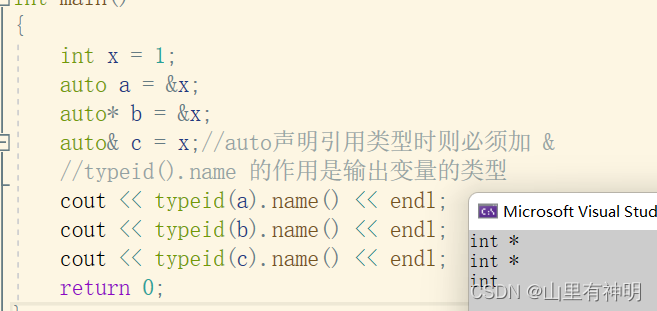### 9.2.2  在同一行定义多个变量

ps：

``````#include<iostream>
using namespace std;
int main()
{
auto a = 1, b = 2;
auto c = 3, d = 4.0; // 该行代码会编译失败，因为c和d的初始化表达式类型不同
return 0;
}``````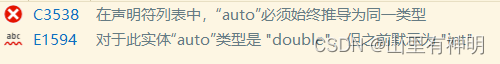## 9.3  auto不能推导的场景

### 9.3.1  auto不能作为函数的参数

``````// 此处代码编译失败，auto不能作为形参类型，因为编译器无法对a的实际类型进行推导
void TestAuto(auto a)
{}``````

### 9.3.2  auto不能直接用来声明数组

ps：

``````#include<iostream>
using namespace std;
int main()
{
int a[] = { 1,2,3 };
auto b[] = a;
return 0;
}``````### 9.3.3

为了避免与 C++ 98中的auto发生混淆，C++11只保留了 auto 作为类型指示符的用法。

### 9.3.4

auto在实际中最常见的优势用法就是跟以后会讲到的C++11提供的新式for循环，还有lambda表达式等进行配合使用。

# 十、基于范围的for循环(C++11)

## 10.1  范围for的语法

``````void TestFor()
{
int array[] = { 1, 2, 3, 4, 5 };
for (int i = 0; i < sizeof(array) / sizeof(array); ++i)
array[i] *= 2;

for (int* p = array; p < array + sizeof(array)/ sizeof(array); ++p)
cout << *p << endl;
}``````

``````void TestFor()
{
int array[] = { 1, 2, 3, 4, 5 };
for(auto& e : array)
e *= 2;

for(auto e : array)
cout << e << " ";

return 0;
}``````

## 10.2  范围for的使用条件

### 10.2.1  for循环迭代的范围必须是确定的

``````void TestFor(int array[])
{
for(auto& e : array)//这里的array 不是数组，数组传参其实是指针传过来
cout<< e <<endl;
}``````

### 10.2.2  迭代的对象要实现 ++ 和 == 的操作

（关于迭代器这个问题，以后会讲，现在提一下，没办法讲清楚，现在大家了解一下就可以了)

# 十一、指针空值nullptr(C++11)

## 11.1  C++98中的指针空值

``````void TestPtr()
{
int* p1 = NULL;
int* p2 = 0;
// ……
}``````

NULL实际是一个宏，在传统的C头文件 (stddef.h) 中，可以看到如下代码：

``````#ifndef NULL
#ifdef __cplusplus
#define NULL 0
#else
#define NULL ((void *)0)
#endif
#endif``````

``````#include<iostream>
using namespace std;
void func(int)
{
cout << "func(int)" << endl;
}
void func(int*)
{
cout << "func(int*)" << endl;
}
int main()
{
func(0);
func(NULL);
func((int*)NULL);
return 0;
}``````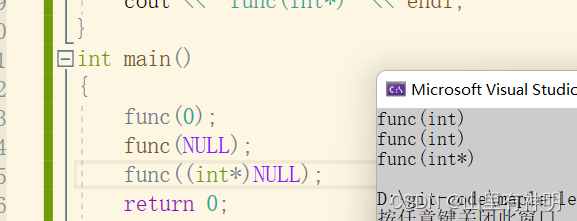在 C++98 中，字面常量0既可以是一个整形数字，也可以是无类型的指针 (void*) 常量，但是编译器默认情况下将其看成是一个整形常量，如果要将其按照指针方式来使用，必须对其进行强转 (void *)0。

## 11.2  C++11中的指针空值nullptr

1. 在使用nullptr表示指针空值时，不需要包含头文件，因为 nullptr 是 C++11 作为新关键字引入的。
2. 在 C++11 中，sizeof(nullptr) 与 sizeof((void*)0) 所占的字节数相同。
3. 为了提高代码的健壮性，在后续表示指针空值时建议最好使用 nullptr。

文章到此结束！